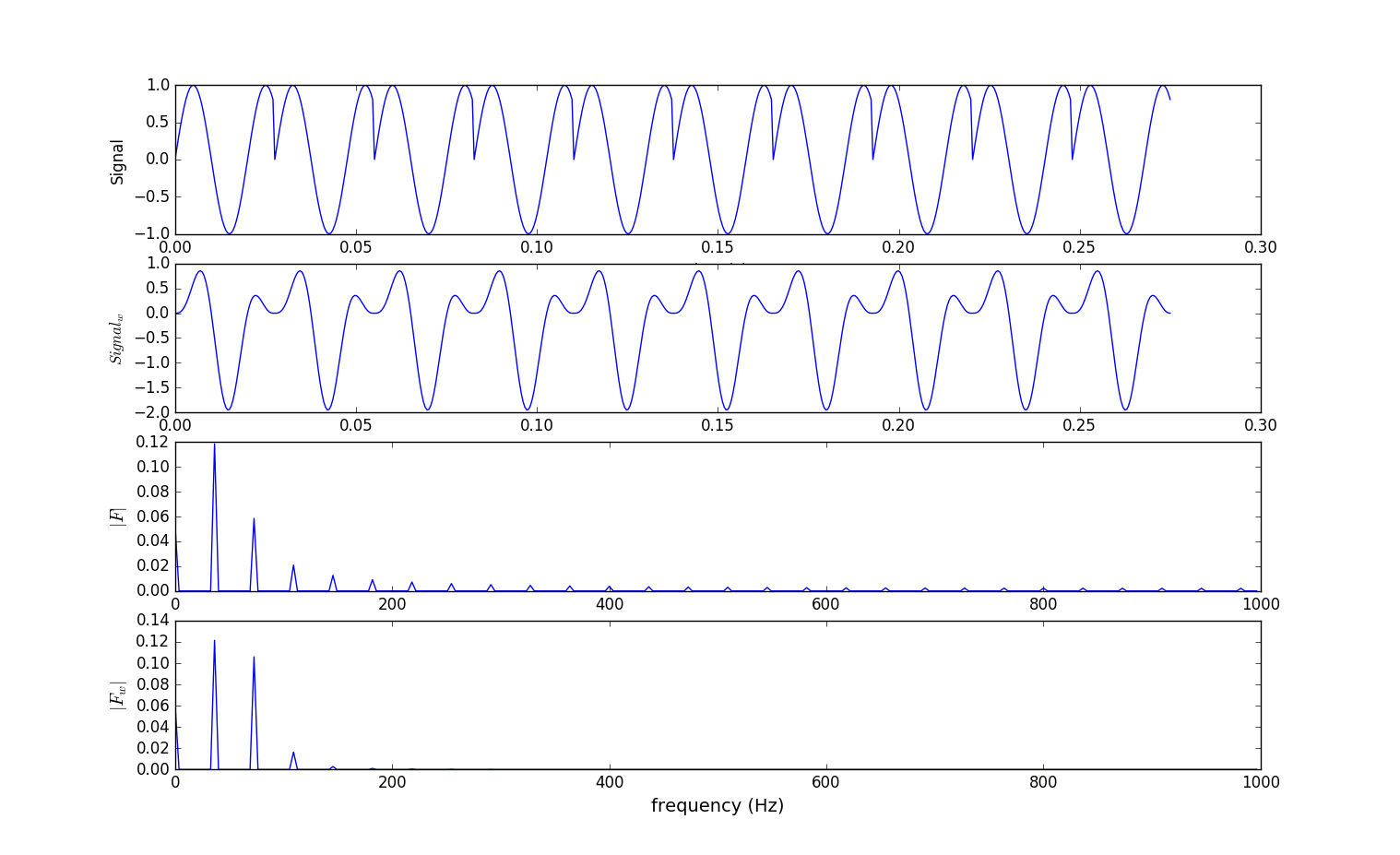# 17. Scipy Tutorial- 非整周期信号加窗

## 17.1 什么是加窗？

#coding:utf-8
import numpy as np
import pylab as pl
from scipy import fftpack,signal
import matplotlib.pyplot as plt
sampling_rate = 100
f_s = 2 * sampling_rate
T = 1
t = np.linspace(0, T, f_s)
x = np.sin(2 * np.pi * 10 * t)
F = fftpack.fft(x)
f = fftpack.fftfreq(T * f_s, 1.0/f_s)
fig, axes = plt.subplots(2, 1, figsize=(8, 6))
axes.plot(t, x, label="Oringal")
axes.set_xlabel("times (s)", fontsize=14)
axes.set_xlabel("frequency (Hz)", fontsize=14)
plt.show()


• 采集到的周期信号完整性和个数对fft的影响

#coding:utf-8
import numpy as np
import pylab as pl
from scipy import fftpack,signal
import matplotlib.pyplot as plt
sampling_rate = 100
f_s = 2 * sampling_rate
T = np.arange(1,21) * 0.05
def drawT(T):
print "t", T
t = np.linspace(0, T, T * f_s)
x = np.sin(2 * np.pi * 10 * t)
F = fftpack.fft(x)
f = fftpack.fftfreq(int(T * f_s), 1.0/f_s)
fig, axes = plt.subplots(2, 1, figsize=(8, 6))
axes.plot(t, x, label="Oringal")
axes.set_xlabel("times (s)", fontsize=14)
axes.set_xlabel("frequency (Hz)", fontsize=14)
plt.show()
for ti in T:
drawT(ti)
print "end"



(1).这个采集了0.05s得到半个$f = sin(10 * 2\pi t)$正弦波得到的结果图：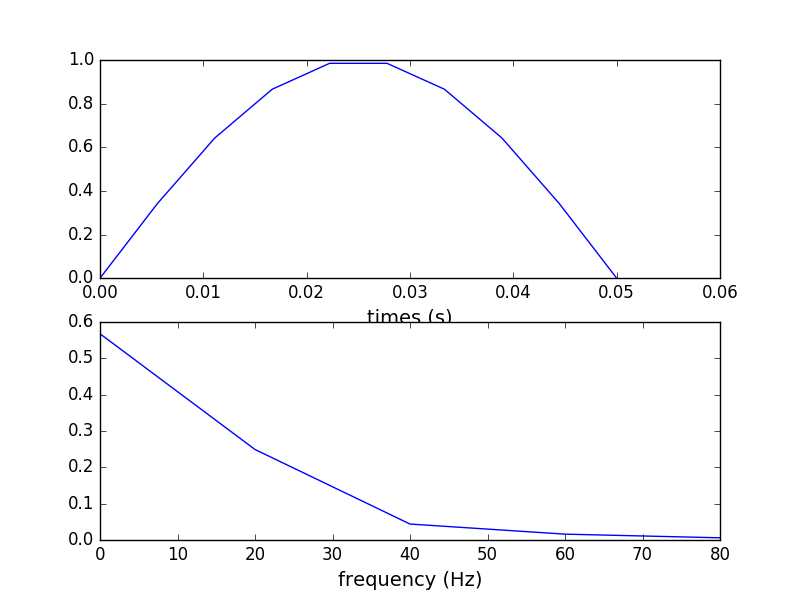这个结果基本看不出啥内容，频率很不好！

(2).采集0.1s得到一个完整的$f = sin(10 * 2\pi t)$正弦波进行fft分析的结果：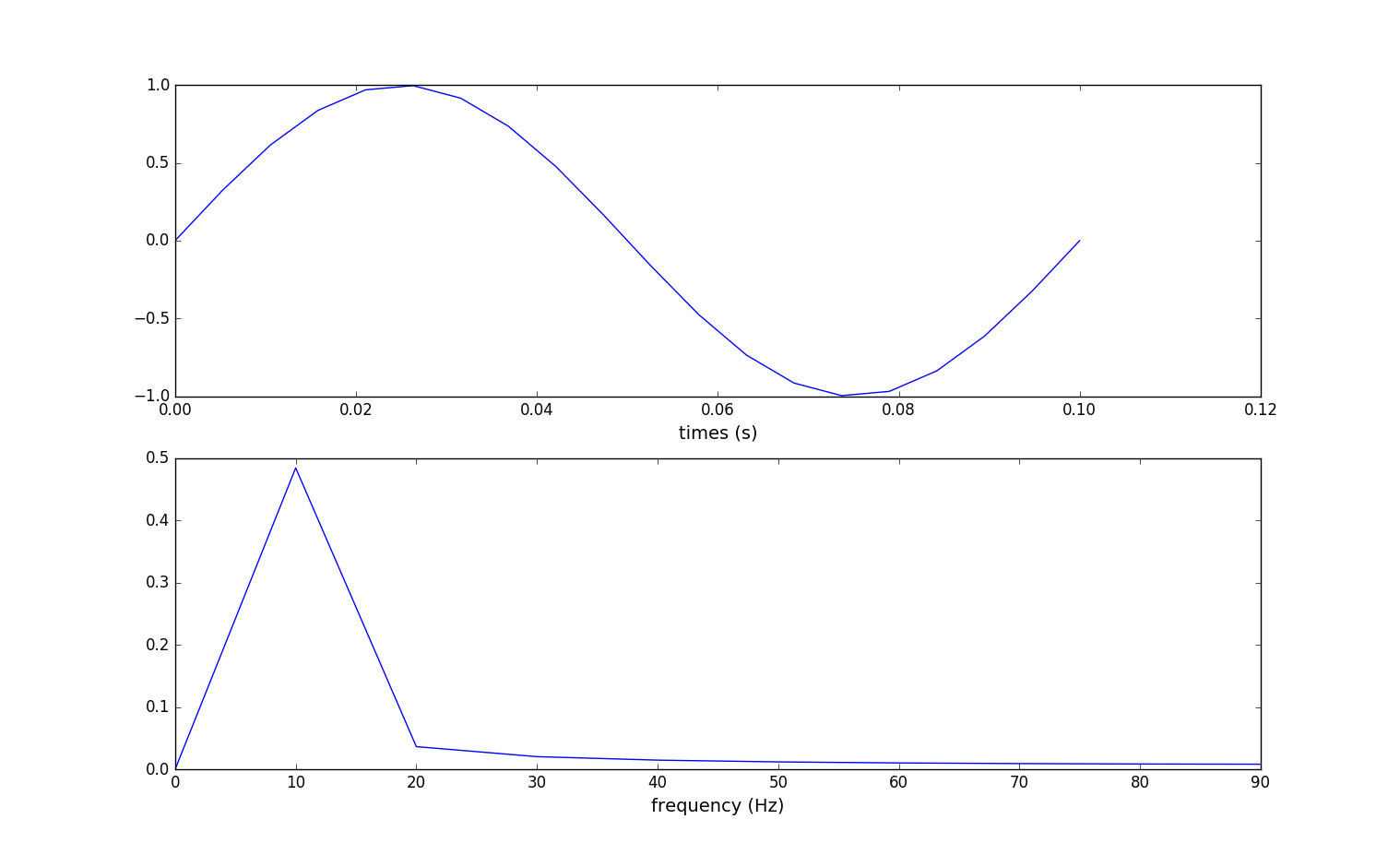结果好点儿。能看出频峰出现在$10Hz$的意思了。

(3).采集0.15s可以得到1个半的正弦波，结果：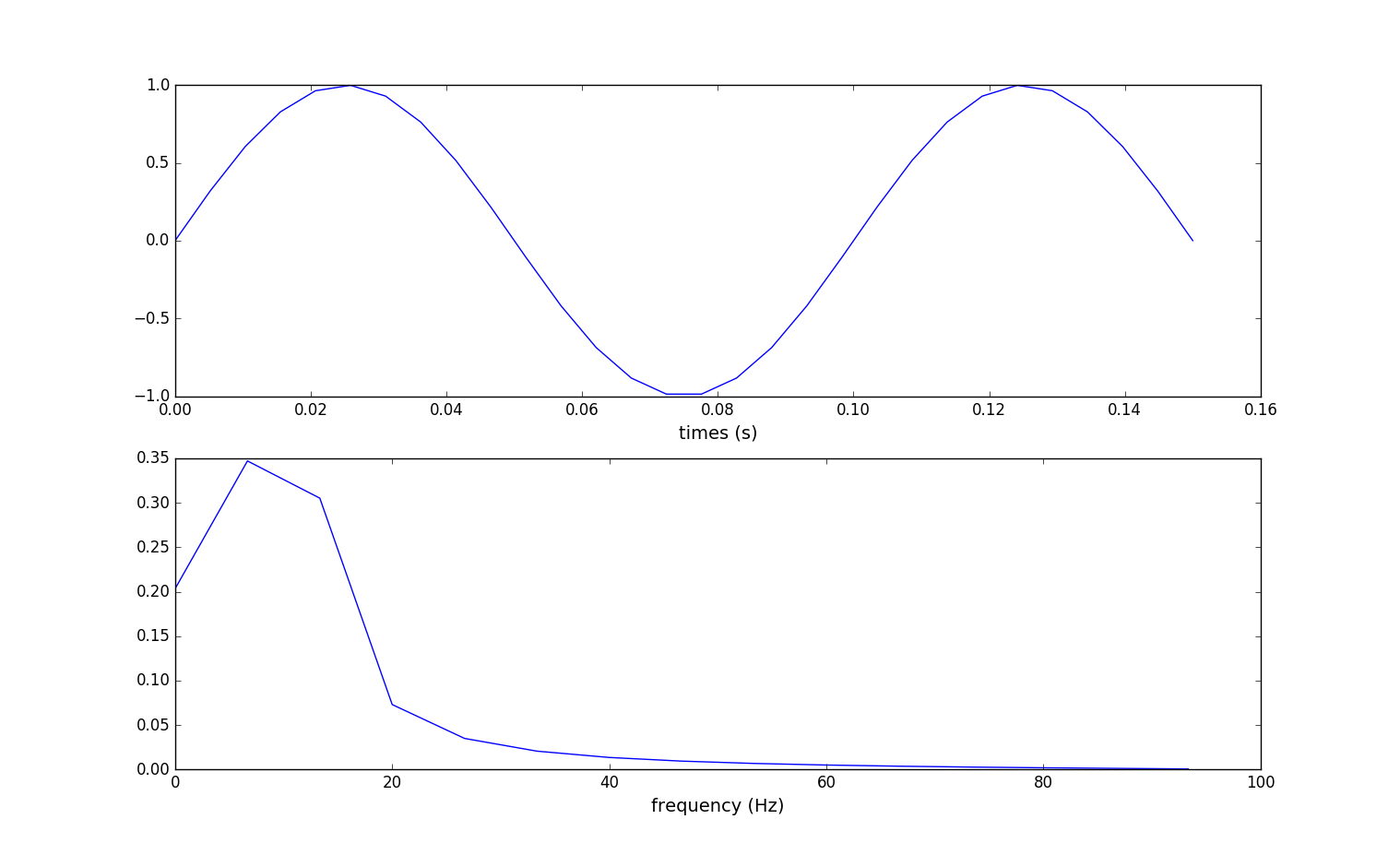结果不完美

(4).采集0.2s得到两个完整正弦波，进行fft处理后的结果图：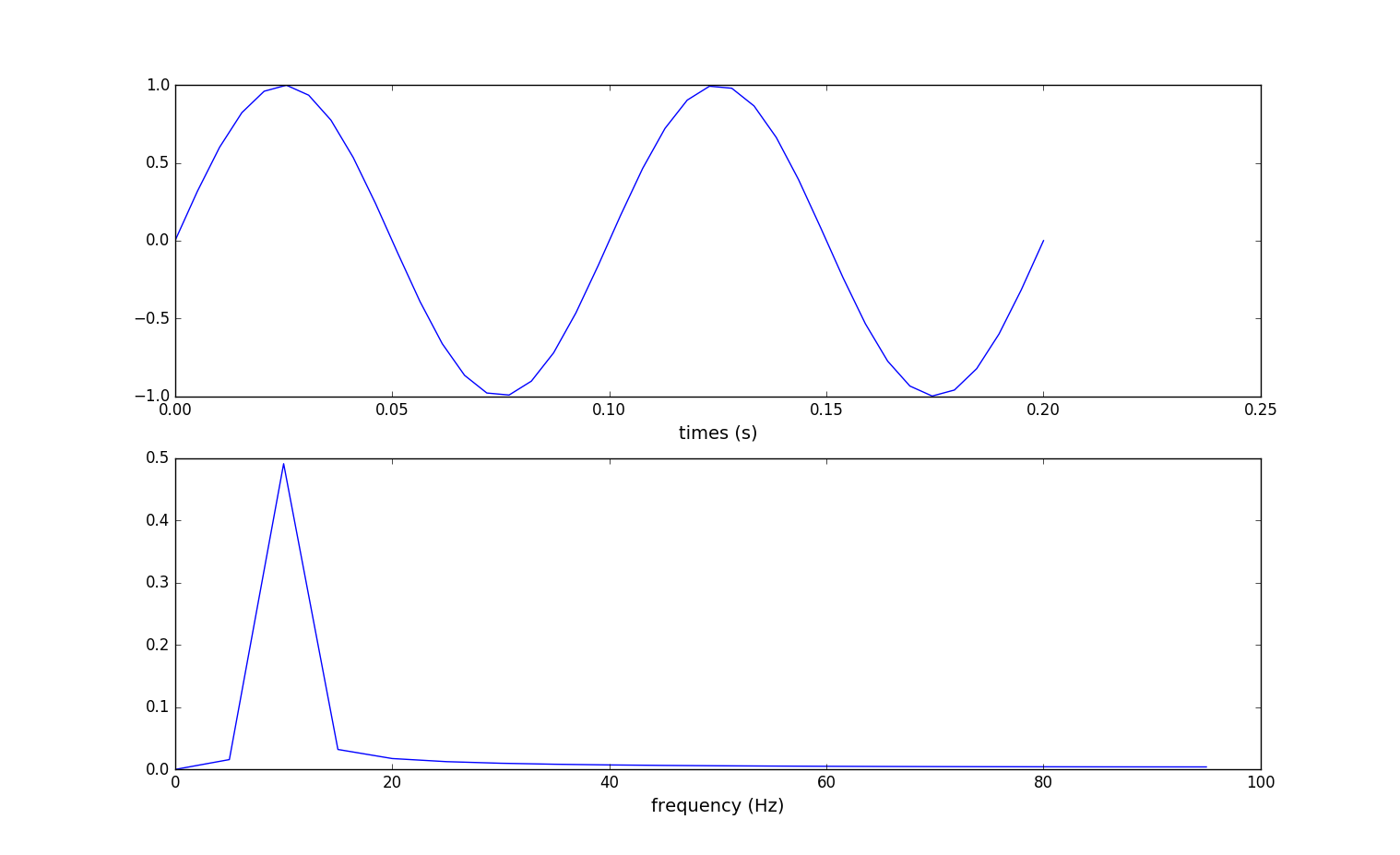结果比01.s的更加完美。

(5).采集0.4s得到四个完整正弦波，进行fft处理后的结果图：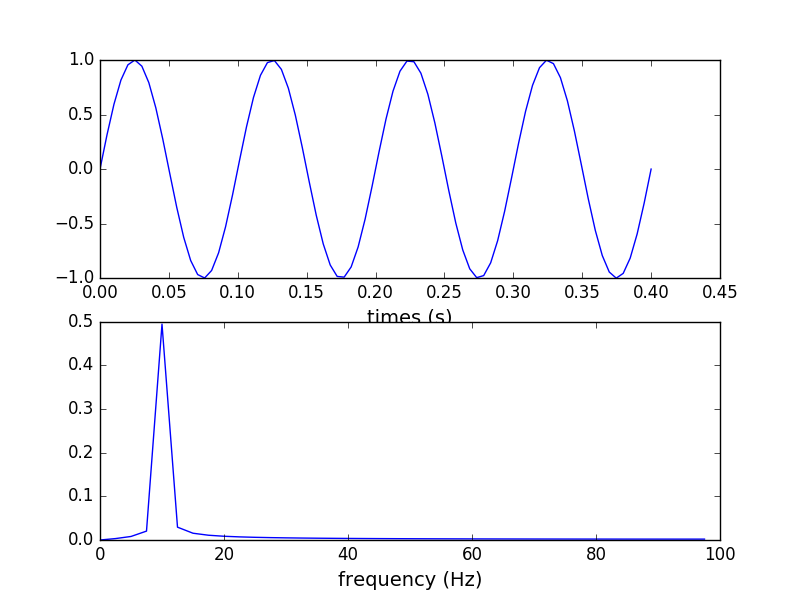结果比02.s的更加完美，频峰出现在$10Hz$很明显。

(6).采集了0.45s的四个半正弦波，结果又不完美了。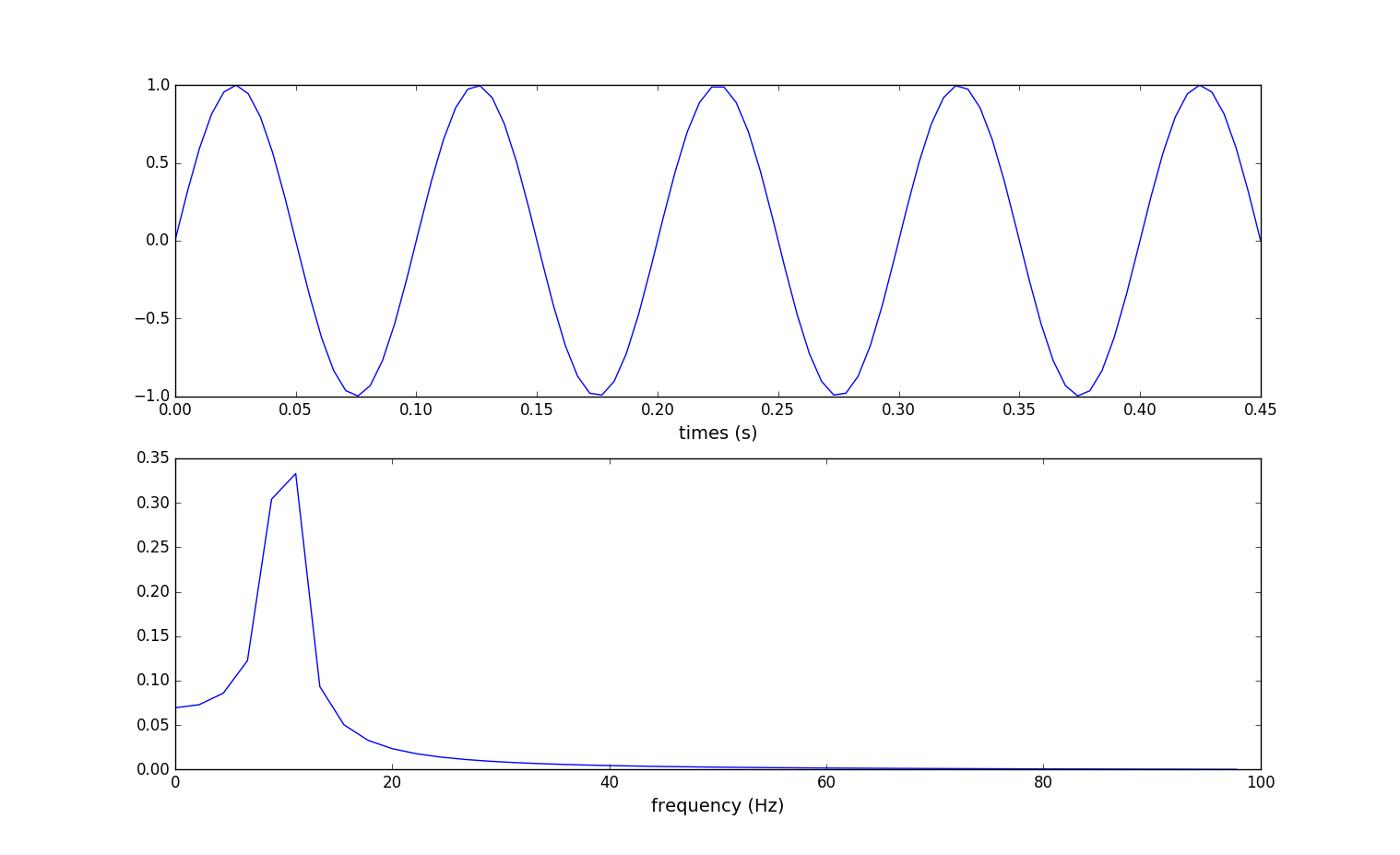随着程序的不断执行。

(7).采集了1s的数据共10个完整的正弦波，经fft处理：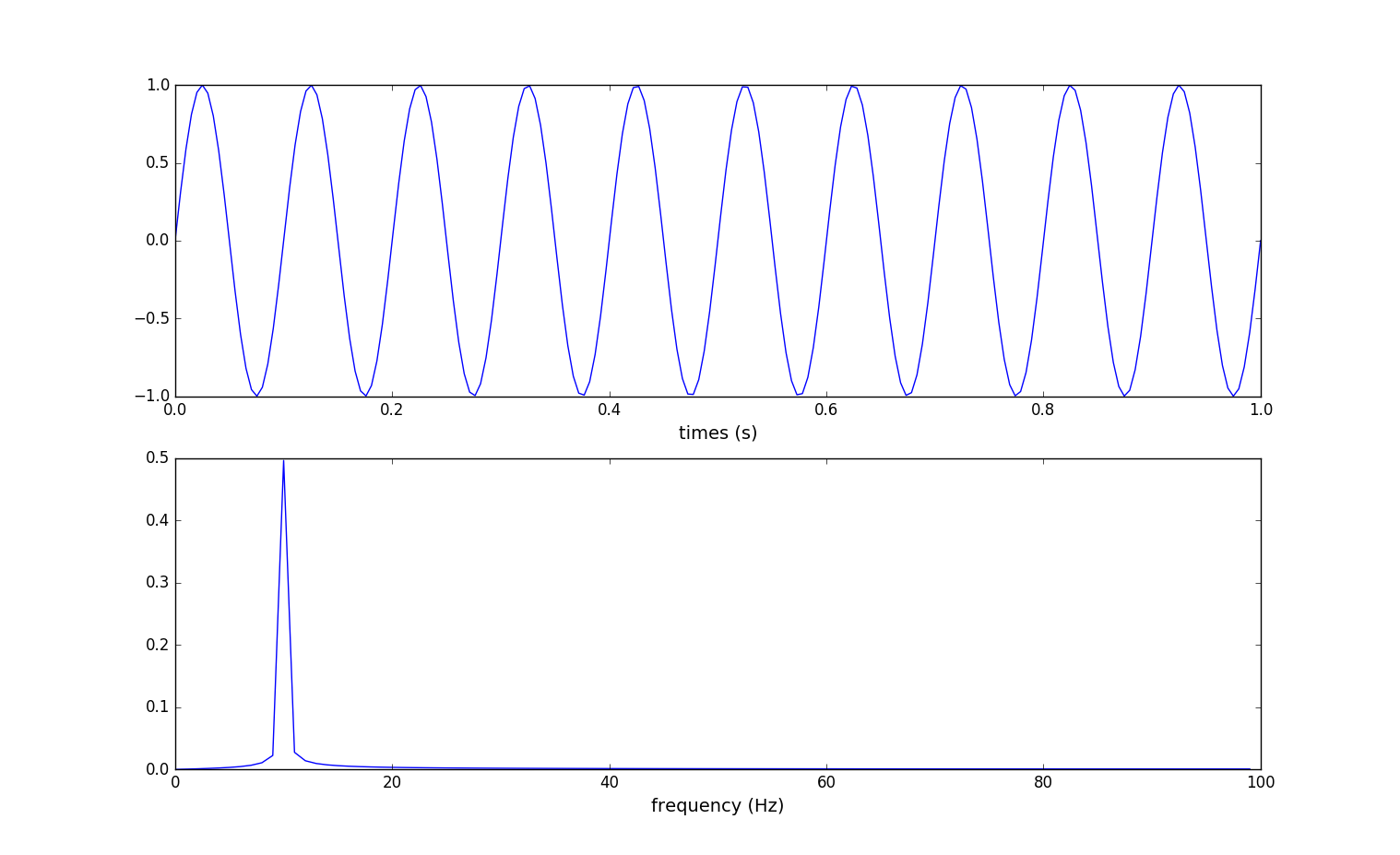结果比之前的都完美，频峰出现在$10Hz$非常明显。

• 不完整的周期信号对fft的影响

#coding:utf-8
import numpy as np
from scipy import fftpack,signal
import matplotlib.pyplot as plt
sampling_rate = 1000
f_s = 2 * sampling_rate
N = 80
T = 1
t = np.linspace(0, T, f_s)[:N]
x = np.sin(2 * np.pi * 50 * t)[:N]

t10 = np.linspace(0, 10 * T * N * 1.0 / f_s, 10 * f_s * N * 1.0 / f_s)
x10 = np.tile(x, 10)

F = fftpack.fft(x10)
f = fftpack.fftfreq(10 * N, 1.0/f_s)
fig, axes = plt.subplots(3,1)
axes.plot(t, x)
axes.set_xlabel("time (s)")
axes.set_ylabel("signal")
axes.plot(t10, x10)
axes.set_xlabel("time (s)")
axes.set_ylabel("signal")
axes.set_xlabel("Frequency (Hz)")
axes.set_ylabel("F")
plt.show()上图最下的子图，比较完美，就一个频峰，大致$50Hz$处出现的。 为何？采样率2000Hz，1s采集2000条数据，正弦波的频率是50Hz那么1s会有50个正弦波，每个正弦波有40条数据，那么80条数据(语句x = np.sin(2 * np.pi * 50 * t)[:N])即$N = 80$会采样得到几个正弦波呢？ $$n = \frac{80}{\frac{2000}{50}} = \frac{80}{40} = 2$$ 得到2个完整的正弦波，即子图1。将80个数据的2个正弦波重复10次(语句x10 = np.tile(x, 10))得到子图2，便可进行fft处理了，得到子图3。. (2).非完整周期信号，程序里只需将N由80改为55个数据点。

#coding:utf-8
#coding:utf-8
import numpy as np
from scipy import fftpack,signal
import matplotlib.pyplot as plt
sampling_rate = 1000
f_s = 2 * sampling_rate
N = 55
T = 1
t = np.linspace(0, T, f_s)[:N]
x = np.sin(2 * np.pi * 50 * t)[:N]

t10 = np.linspace(0, 10 * T * N * 1.0 / f_s, 10 * f_s * N * 1.0 / f_s)
x10 = np.tile(x, 10)

F = fftpack.fft(x10)
f = fftpack.fftfreq(10 * N, 1.0/f_s)
fig, axes = plt.subplots(3,1)
axes.plot(t, x)
axes.set_xlabel("time (s)")
axes.set_ylabel("signal")
axes.plot(t10, x10)
axes.set_xlabel("time (s)")
axes.set_ylabel("signal")
axes.set_xlabel("Frequency (Hz)")
axes.set_ylabel("F")
plt.show()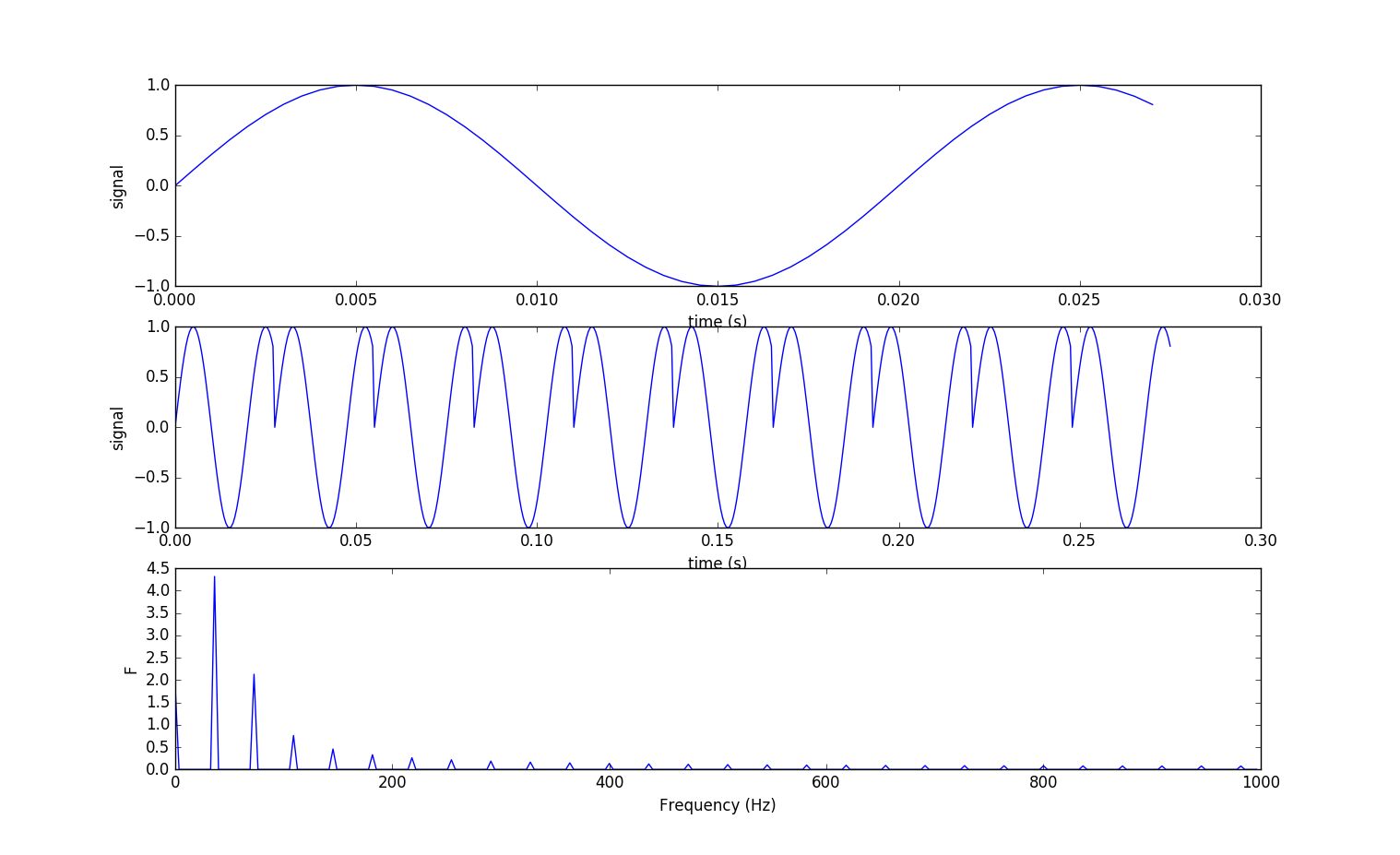这个结果很意外么？对比此图的2、3子图和上一个图的2、3子图，在本图的2子图是非连续的信号(大致在0.025s$\sim$0.3s间)，所以在本图的3子图里出现了很多的频峰，能量被分散到其他频率里去了。之后使用FFT获得的频率，不是原信号的实际频率，而是一个改变过的频率。 类似于某个频率的能量泄漏至其他频率，这种现象叫做频谱泄漏。频率泄漏使好的频谱线扩散到更宽的信号范围中。谱泄漏对频谱图的影响的大小取决于时域图中边界上的不连续程度。加窗方法可以将这种不连续最小化。窗函数实质上是一个加权函数。通过加窗函数与原始函数相乘，改善能量泄露的状况。 程序里$N = 55$只是截取了 $$n = \frac{55}{\frac{2000}{50}} = \frac{55}{40} = 1 \frac{3}{8}$$ 个正弦波(语句x = np.sin(2 * np.pi * 50 * t)[:N])，见子图1。重复10次(见语句x10 = np.tile(x, 10))，见子图2。fft分析里有很多频率来源于子图2里的不连续点(大致在0.025s$\sim$0.3s间)。如何解决？阅读下一节。

## 17.2 加窗函数

(1).如果您的信号具有强干扰频率分量，与感兴趣分量相距较远，那么就应选择具有高旁瓣下降率的平滑窗。 (2).如果您的信号具有强干扰频率分量，与感兴趣分量相距较近，那么就应选择具有低最大旁瓣的窗。 (3).如果感兴趣频率包含两种或多种很距离很近的信号，这时频谱分辨率就非常重要。 在这种情况下，最好选用具有窄主瓣的平滑窗。 (4).如果一个频率成分的幅值精度比信号成分在某个频率区间内精确位置更重要，选择宽主瓣的窗。 (5).如信号频谱较平或频率成分较宽，使用统一窗，或不使用窗。 总之，Hanning窗适用于95%的情况。 它不仅具有较好的频率分辨率，还可减少频谱泄露。 如果您不知道信号特征但是又想使用平滑窗，那么就选择Hanning窗。

Hamming窗和Hanning窗都有正弦波的外形。 两个窗都会产生宽波峰低旁瓣的结果。 Hanning窗在窗口的两端都为0，杜绝了所有不连续性。 Hamming窗的窗口两端不为0，信号中仍然会呈现不连续性。 Hamming窗擅长减少最近的旁瓣，但是不擅长减少其他旁瓣。 Hamming窗和Hanning适用于对频率精度要求较高对旁瓣要求较低的噪声测量。

 #coding:utf-8
import numpy as np
from scipy import fftpack,signal
import matplotlib.pyplot as plt
sampling_rate = 1000
f_s = 2 * sampling_rate
N = 55
T = 1
t = np.linspace(0, T, f_s)[:N]
x = np.sin(2 * np.pi * 50 * t)[:N]

t10 = np.linspace(0, 10 * T * N * 1.0 / f_s, 10 * f_s * N * 1.0 / f_s)
x10 = np.tile(x, 10)

F = fftpack.fft(x10)
f = fftpack.fftfreq(10 * N, 1.0/f_s)
fig, axes = plt.subplots(3,1)
axes.plot(t, x)
axes.set_xlabel("time (s)")
axes.set_ylabel("signal")
axes.plot(t10, x10)
axes.set_xlabel("time (s)")
axes.set_ylabel("signal")
axes.set_xlabel("time (s)")
axes.set_ylabel("signal")
plt.show()

from scipy import fftpack,signal
window = signal.hann(len(x), sym = 0) * 2
x10w = np.tile(x * window, 10)
F_w = fftpack.fft(x10w)
F = fftpack.fft(x10)
f = fftpack.fftfreq(10 * N, 1.0/f_s)
fig, axes = plt.subplots(4, 1, figsize=(8, 6))

axes.plot(t10, x10)
axes.set_xlabel("time (s)")
axes.set_ylabel("Signal")
axes.plot(t10, x10w, label="real")
axes.set_ylabel("$Signal_w$")
axes.set_ylabel("$|F|$", fontsize=14)
axes.set_xlabel("frequency (Hz)", fontsize=14)
axes.set_ylabel("$|F_w|$", fontsize=14)
plt.show()


window = signal.hann(len(x), sym = 0) * 2 #信号加窗
x10w = np.tile(x * window, 10) #10倍扩展重复


F_w = fftpack.fft(x10w)
F = fftpack.fft(x10)